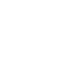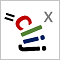# CLIJ2GPU accelerated image processing for everyone

CLIJ2 home

## generateGreyValueCooccurrenceMatrixBoxTakes an image and an intensity range to determine a grey value co-occurrence matrix.

For determining which pixel intensities are neighbors, the box neighborhood is taken into account. Pixels with intensity below minimum of the given range are considered having the minimum intensity. Pixels with intensity above the maximimum of the given range are treated analogously. The resulting co-occurrence matrix contains probability values between 0 and 1.

Category: Measurements

Availability: Available in Fiji by activating the update sites clij and clij2. This function is part of clijx_-0.32.0.1.jar.

### Usage in ImageJ macro

``````Ext.CLIJx_generateGreyValueCooccurrenceMatrixBox(Image integer_image, Image grey_value_cooccurrence_matrix_destination, Number min_grey_value, Number max_grey_value);
``````

### Usage in object oriented programming languages

Java
```// init CLIJ and GPU
import net.haesleinhuepf.clijx.CLIJx;
import net.haesleinhuepf.clij.clearcl.ClearCLBuffer;
CLIJx clijx = CLIJx.getInstance();

// get input parameters
ClearCLBuffer integer_image = clijx.push(integer_imageImagePlus);
grey_value_cooccurrence_matrix_destination = clijx.create(integer_image);
float min_grey_value = 1.0;
float max_grey_value = 2.0;
```
```// Execute operation on GPU
clijx.generateGreyValueCooccurrenceMatrixBox(integer_image, grey_value_cooccurrence_matrix_destination, min_grey_value, max_grey_value);
```
```// show result
grey_value_cooccurrence_matrix_destinationImagePlus = clijx.pull(grey_value_cooccurrence_matrix_destination);
grey_value_cooccurrence_matrix_destinationImagePlus.show();

// cleanup memory on GPU
clijx.release(integer_image);
clijx.release(grey_value_cooccurrence_matrix_destination);
```
Matlab
```% init CLIJ and GPU
clijx = init_clatlabx();

% get input parameters
integer_image = clijx.pushMat(integer_image_matrix);
grey_value_cooccurrence_matrix_destination = clijx.create(integer_image);
min_grey_value = 1.0;
max_grey_value = 2.0;
```
```% Execute operation on GPU
clijx.generateGreyValueCooccurrenceMatrixBox(integer_image, grey_value_cooccurrence_matrix_destination, min_grey_value, max_grey_value);
```
```% show result
grey_value_cooccurrence_matrix_destination = clijx.pullMat(grey_value_cooccurrence_matrix_destination)

% cleanup memory on GPU
clijx.release(integer_image);
clijx.release(grey_value_cooccurrence_matrix_destination);
```

Imprint